Ratio, proportion and percentages

This free course is available to start right now. Review the full course description and key learning outcomes and create an account and enrol if you want a free statement of participation.

Free course

1.4 Converting ratios from fractions to decimals

Although ratios are often given as fractions, they can also be expressed as decimals. You need to deal with a mixture of fractions and decimals, and to compare ratios given in either form, so you need to be able to convert between the two forms.

Example 4

The ratio of the circumference of a circle to its diameter is a constant denoted by(the Greek letter p) pronounced pi, it has been approximated by a number of different fractions. One such fraction is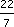, another is 355/113. How do these compare with the decimal value from a calculator of 3.141592 654?

If you have a calculator handy then you could key in 22 ÷ 7 to convert to a decimal. However, if not, you might use long division or an informal method of division. Either way you should get 3.1428 …. Soagrees to 3 significant figures.

You will probably find it easier to use a calculator for dividing 355 by 113. The result is 3.14159292, which agrees to 7 significant figures.

Sometimes you are given a ratio as a decimal, but might find it easier to use and/or remember as a fraction.

Example 5

Suppose you had been told that the ratio between a distance measured in miles and the same distance measured in kilometres is about 0.625. Convert this to a fraction.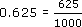This fraction is an adequate answer, or you can divide top and bottom by common factors to reach a simpler equivalent fraction.

Divide top and bottom by 5 to get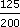.

Divide top and bottom by 5 again to get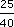.

Divide top and bottom by 5 again to get.

So the fraction is, which is the fraction used earlier.

An alternative method is do this in one line cancelling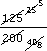MU120_4M3